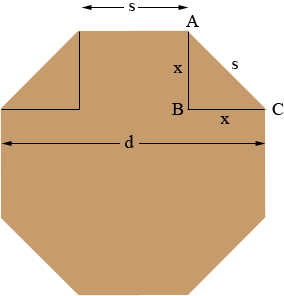SEARCH HOMEMath Central Quandaries & QueriesQuestion from mark, a parent: I need to know the measurements of an octagon with a inside diameter of 34.5 inches from side to sideHi Mark,

Suppose the distance from side to side of a regular octagon is d units and the length of a side is s units.From the diagram d = s + 2x. Triangle ABC is a right triangle so Pythagoras theorem states that

s2 = x2 + x2 = 2x2

or

x = s/√2

Thus

d = s + 2x = s + 2s/√2 = s(1 + 2/√2) = (1 + √2) s

or

s = 1/(1 + √2) d

PennyMath Central is supported by the University of Regina and The Pacific Institute for the Mathematical Sciences.